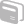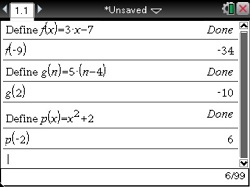# Activities

••• ##### Subject Area

• Math: Algebra I: Equivalence

• ##### Author9-12

45 Minutes

• ##### Device
• TI-Nspire™
• TI-Nspire™ CAS

2.1

## Computing with Mathematical Formulas#### Activity Overview

Evaluate formulas for given values of a variable.

#### Objectives

• Evaluate any algebraic expression for real number values of its variables
• Use technology to verify the evaluation of any algebraic expression for real values of its variables